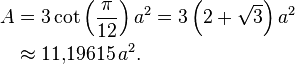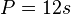# Dodecagon facts for kids

Kids Encyclopedia Facts

A dodecagon or 12-gon is a shape with 12 sides and 12 corners.

## Regular dodecagon

All sides of a regular dodecagon are the same length. Each corner is 150°. All corners added together equal 1800°.

### Area

The amount of space a regular dodecagon takes up is\begin{align} A & = 3 \cot\left(\frac{\pi}{12} \right) a^2 = 3 \left(2+\sqrt{3} \right) a^2 \\ & \approx 11{,}19615\,a^2. \end{align}
The variable A represents the area, and the variable a represents the apothem.

### Perimeter

The perimeter of a regular dodecagon is\begin{align} P = 12s \end{align}

The variable P represents the perimeter, and the variable s represents one side.

## Images for kidsDodecagon Facts for Kids. Kiddle Encyclopedia.